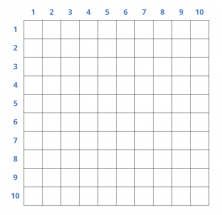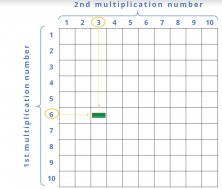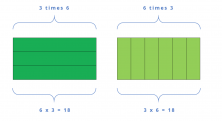Smartick is a fun way to learn math!May04

# Learn New Tricks to Memorize Multiplication Tables!

### Playing with Multiplication Tables

In today’s post we’re going to learn a game to help memorize multiplication tables in a fun, understandable, and visual way.

We know that memorizing multiplication tables increases our calculation skills, but most importantly, especially during the first few years we start learning them, it helps us understand the process of calculation going on behind them.

The following game will be very useful for this process.

### Materials

The first thing you’ll need is a box of Cuisenaire rods. If you have them in the house, great, if not, you can download this pdf so you can make some out of paper and play with them (if you can mold them out of clay or something similar, even better!).

You will also need 2 dice numbered 0 to 5 (0, 1, 2, 3, 4, and 5). If you want, you can make them out of cardboard, or use normal dice by writing a zero on two stickers and covering the sixes with them. You can also use the dice as they are, and remember not to add anything when you roll a 6.

Finally, you will need a grid made up of 10 rows and 10 columns, that you can make using craft paper, or draw one on the floor outside using tape or chalk (it will look nicer if you use different colors). You should have something like this:### Rules

Once you’ve got your materials, you only need to learn the rules before you can start playing. They are the following:

• Roll the dice and add the numbers that you get. The sum (between 0 and 10) will be the first number we use for the multiplication problem. If you get a 0, roll again. Let’s imagine our first number is 6.
• Roll the dice again to get the second number for the multiplication problem. Imagine that our second number is 3.
• Calculate the result of the multiplication problem. In this example, since we’ve got a 6 and 3, we would have to multiply 6 x 3 = 18.
• Construct the multiplication problem with the Cuisenaire rods. In our example, we would have to construct the multiplication 6 x 3. To do this, we take 3 rods of 6 and put them in the corresponding square, like this:• Check the result of the multiplication problem. Line the rods up. Next, place the largest rod you can alongside them (for example 10), and keep placing rods until the two lengths match.• If the result of adding the rods that match yours equals the result of your multiplication, you win a point!

You can play alone or with friends until there are no rods left. The person who scores the most points is the winner.

### Constructing multiplication tables with rods

If you have enough rods, you can also construct a multiplication table, alone or with a friend, without having to roll dice or take turns.

You’ll be able to see some really interesting things, like the commutative property of the multiplication, since 6 x 3 will occupy the same amount of space as 3 x 6:If you like this game, share it with your friends so they can play and have fun learning their multiplication tables too!